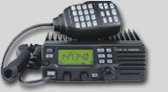# Amateur Beam Antenna Calculator Three Element Yagi

Here is a simple Javascript calculator for determining the lengths of tubing to be used for the construction of a Super J-Pole Collinear Design. (Note: It is always good practice to use an Antenna Analyzer when building a custom antenna!)

 Designation Number Required Data Entry Desired Frequency Mhz Calculated Results Calculated Driven Side Element Length Calculated Total Driven Length Calculated Reflector Length Calculated Element Spacing Calculated Director Length

This calculator is designed to give the critical information of a particular beam antenna, in this case a three element Yagi, for the frequency chosen. Enter the desired frequency then click on Calculate and the optimum values for that combination will be displayed in feet, inches and fractions of inches, and in meters. To optimize the antenna for a frequency RANGE, do the calculations twice, once for the low end of the range and once for the high end; then average the two and plan to adjust the VSWR on both ends of the range as needed. As general information, the director is the shortest element, the driven element is the middle element (in a 3 element beam) and the reflector is the longest element. Spacing is on the antenna bar holding the elements, with equidistant spacing from each of the outside elements to the driven element. Direction of the strongest transmitted signal is from the reflector toward the director. Reception is the reverse.Popular Callsign Lookups
for this month:

WA9TTV
N4HHP
N8UKC
WW4ASA
KB6LWP